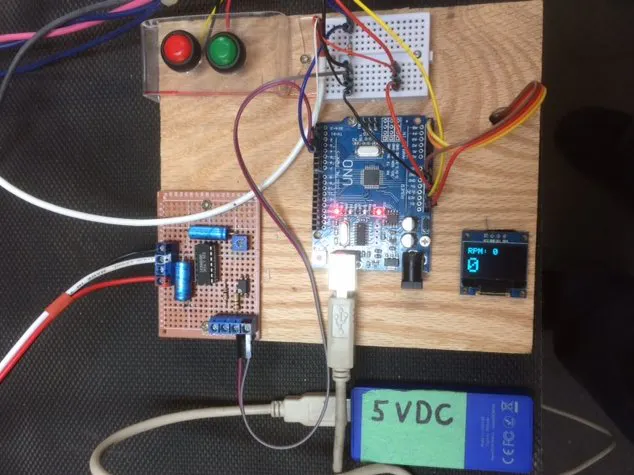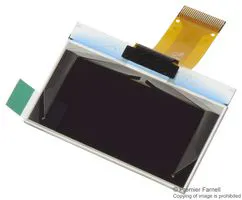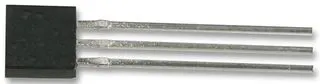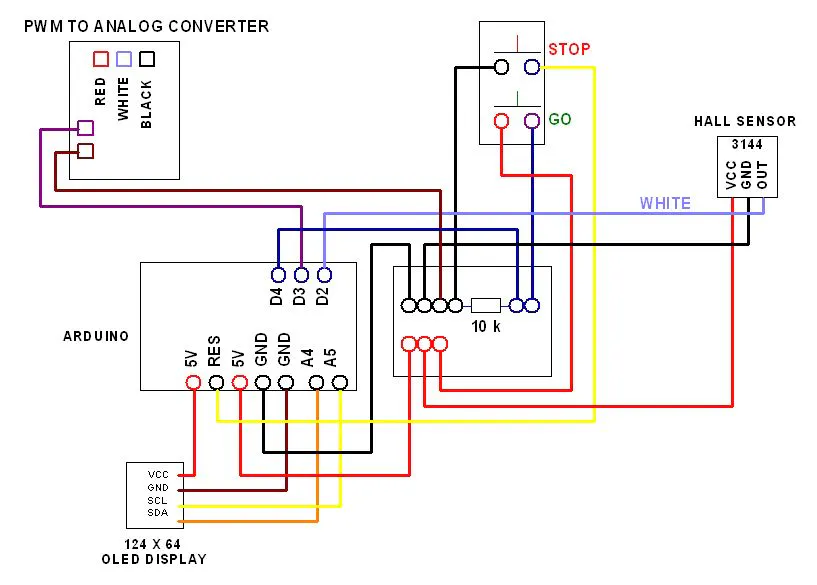# Incremental Speed Control of a Treadmill Motor

An inexpensive project that I put together to set the RPM of a treadmill motor at five preset speeds.

IntermediateFull instructions provided1,501## Things used in this project

### Hardware componentsArduino UNO & Genuino UNO
×1Graphic OLED, 128 x 64
×1Hall Effect Sensor I used a 3144 Hall sensor
×1

## Schematics

### Wiring Layout

A wiring layout for this project more information is provided in my video.## Code

C/C++
It is a incremental speed controller for a treadmill motor, five previously set speeds are selected using a single push button.
`No preview (download only).`

C/C++
```/*
Tachometer using micros

On this sketch we are going to measure the period between
pulses using the micros() function to get the RPM
(Revolutions Per Minute) from a sensor on pin 2.
This way of measuring RPM makes it accurate, responsive and
versatile. No matter how fast or slow the loop is
running, the reading accuracy is not going to be affected.
Although, the faster you run the loop, the more amount
of readings you are going to be able to display every
second.

It's coded in a way that the micros rollover doesn't create
glitches every 71 minutes, so it can run forever
without problems.

We use an interrupt for the input so you have to choose pin
2 or 3 (for Arduino Uno/nano). In this example we
use pin 2.

This sketch was made for my video tutorial shown here:

Last update: 05/23/2019
*/

///////////////
// Calibration:
///////////////

const byte PulsesPerRevolution = 1;  // Set how many pulses
there are on each revolution. Default: 2.

// If the period between pulses is too high, or even if the
pulses stopped, then we would get stuck showing the
// last value instead of a 0. Because of this we are going
to set a limit for the maximum period allowed.
// If the period is above this value, the RPM will show as
0.
// The higher the set value, the longer lag/delay will have
to sense that pulses stopped, but it will allow readings
// at very low RPM.
// Setting a low value is going to allow the detection of
stop situations faster, but it will prevent having low RPM
// The unit is in microseconds.
const unsigned long ZeroTimeout = 300000;  // For high
response time, a good value would be 100000.
very low RPM, a good value would be 300000.

// Calibration for smoothing RPM:
const byte numReadings = 2;  // Number of samples for
smoothing. The higher, the more smoothing, but it's going
to
// react slower to changes. 1
= no smoothing. Default: 2.

/////////////
// Variables:
/////////////

volatile unsigned long LastTimeWeMeasured;  // Stores the
last time we measured a pulse so we can calculate the
period.
volatile unsigned long PeriodBetweenPulses = ZeroTimeout
+1000;  // Stores the period between pulses in
microseconds.
// It has a big number so it doesn't
frequency.
volatile unsigned long PeriodAverage = ZeroTimeout+1000;
// Stores the period between pulses in microseconds in
total, if we are taking multiple pulses.
// It has a big number so it doesn't
frequency.
unsigned long FrequencyRaw;  // Calculated frequency, based
on the period. This has a lot of extra decimals without the
decimal point.
unsigned long FrequencyReal;  // Frequency without
decimals.
unsigned long RPM;  // Raw RPM without any processing.
unsigned int PulseCounter = 1;  // Counts the amount of
pulse readings we took so we can average multiple pulses
before calculating the period.

unsigned long PeriodSum; // Stores the summation of all the
periods to do the average.

unsigned long LastTimeCycleMeasure = LastTimeWeMeasured;
// Stores the last time we measure a pulse in that cycle.
// We need a variable
with a value that is not going to be affected by the
interrupt
// because we are going
to do math and functions that are going to mess up if the
values
// changes in the
middle of the cycle.
unsigned long CurrentMicros = micros();  // Stores the
micros in that cycle.
// We need a
variable with a value that is not going to be affected by
the interrupt
// because we are
going to do math and functions that are going to mess up if
the values
// changes in the
middle of the cycle.

// We get the RPM by measuring the time between 2 or more
pulses so the following will set how many pulses to
// take before calculating the RPM. 1 would be the minimum
giving a result every pulse, which would feel very
responsive
// even at very low speeds but also is going to be less
accurate at higher speeds.
// With a value around 10 you will get a very accurate
result at high speeds, but readings at lower speeds are
going to be
// farther from eachother making it less "real time" at
those speeds.
// There's a function that will set the value depending on
the speed so this is done automatically.
#include <Wire.h>

#define OLED_WIDTH 128
#define OLED_HEIGHT 64

unsigned int ZeroDebouncingExtra;  // Stores the extra
value added to the ZeroTimeout to debounce it.
// The ZeroTimeout needs
debouncing so when the value is close to the threshold it
// doesn't jump from 0
to the value. This extra value changes the threshold a
little
// when we show a 0.

// Variables for smoothing tachometer:
unsigned long readIndex;  // The index of the current
unsigned long total;  // The running total.
unsigned long average;  // The RPM value after applying the
smoothing.

void isr()          //interrupt service routine
{

}

const int MOTOR = 3;            //Motor connected to
digital pin 3
const int BUTTON = 4;           //Button connected to pin 4
boolean lastButton = LOW;       //Variable containing the
previous
//button state
boolean currentButton = LOW;    //Variable containing the
current
//button state

int speedIncrement = 0;

void setup()  // Start of setup:
{
display.clearDisplay();
digitalWrite (2 ,HIGH);
display.clearDisplay();

digitalWrite(2 ,HIGH);// Instead of using a pull up
resistor
attachInterrupt(0,isr,RISING);  //attaching the interrupt
pinMode (MOTOR, OUTPUT);      //Set the MOTOR pin as an
output
pinMode (BUTTON, INPUT);      //Set button as an input
}
/*
* Debouncing Funtion
* Pass it the previous button state,
* and get back the current debounced button state.
*/
boolean debounce(boolean last)
{
button state
if (last != current)                      //if it's
different
{
delay(5);                             //wait 5ms
}
return current;
}

/*
*Motor Speed Selection
*Pass a number for the Motor speed and set it
*/

void setSpeed(int increment)

{

//speed one
if (increment == 1)

{
analogWrite(MOTOR, 50);//Original values 110 150 180 200
255
display.setTextSize(2);
display.setTextColor(WHITE);
display.setCursor(60, 0);// Vertical, Horizontal.
display.println("*");
display.display();
}

else if (increment == 2)
{
analogWrite(MOTOR, 75);
display.setTextSize(2);
display.setTextColor(WHITE);
display.setCursor(60, 0);// Vertical, Horizontal.
display.println("**");
display.display();
}
else if (increment == 3)
{
analogWrite(MOTOR, 100);
display.setTextSize(2);
display.setTextColor(WHITE);
display.setCursor(60, 0);// Vertical, Horizontal.
display.println("***");
display.display();
}
else if (increment == 4)
{
analogWrite(MOTOR, 125);
display.setTextSize(2);
display.setTextColor(WHITE);
display.setCursor(60, 0);// Vertical, Horizontal.
display.println("****");
display.display();
}
else if (increment == 5)
{
analogWrite(MOTOR, 150);
display.setTextSize(2);
display.setTextColor(WHITE);
display.setCursor(60, 0);// Vertical, Horizontal.
display.println("*****");
display.display();
}

//OFF
else
{
analogWrite(MOTOR, 0);
display.setTextSize(2);
display.setTextColor(WHITE);
display.setCursor(60, 0);// Vertical, Horizontal.
display.println("0");
display.display();
}

attachInterrupt(digitalPinToInterrupt(2), Pulse_Event,
RISING);  // Enable interruption pin 2 when going from LOW
to HIGH.

delay(1000);  // We sometimes take several readings of
the period to average. Since we don't have any readings
// stored we need a high enough value in
micros() so if divided is not going to give negative
values.
// The delay allows the micros() to be high
enough for the first few cycles.

}  // End of setup.

void loop()  // Start of loop:
{

currentButton = debounce(lastButton);
if (lastButton == LOW && currentButton == HIGH)     //if
it was pressed
{
speedIncrement++;
//Increment motor speed
}
lastButton = currentButton;
//reset button value

//if cycled through all speed increments
//reset the speed to 0
if (speedIncrement == 6)  speedIncrement = 0;

setSpeed(speedIncrement);
// The following is going to store the two values that
might change in the middle of the cycle.
// We are going to do math and functions with those
values and they can create glitches if they change in the
// middle of the cycle.
LastTimeCycleMeasure = LastTimeWeMeasured;  // Store the
LastTimeWeMeasured in a variable.
CurrentMicros = micros();  // Store the micros() in a
variable.

// CurrentMicros should always be higher than
LastTimeWeMeasured, but in rare occasions that's not true.
// I'm not sure why this happens, but my solution is to
compare both and if CurrentMicros is lower than
// LastTimeCycleMeasure I set it as the CurrentMicros.
// The need of fixing this is that we later use this
information to see if pulses stopped.
if(CurrentMicros < LastTimeCycleMeasure)
{
LastTimeCycleMeasure = CurrentMicros;
}
// Calculate the frequency:
FrequencyRaw = 10000000000 / PeriodAverage;  // Calculate
the frequency using the period between pulses.

// Detect if pulses stopped or frequency is too low, so
we can show 0 Frequency:
if(PeriodBetweenPulses > ZeroTimeout -
ZeroDebouncingExtra || CurrentMicros - LastTimeCycleMeasure
> ZeroTimeout - ZeroDebouncingExtra)
{  // If the pulses are too far apart that we reached the
timeout for zero:
FrequencyRaw = 0;  // Set frequency as 0.
ZeroDebouncingExtra = 2000;  // Change the threshold a
little so it doesn't bounce.
}
else
{
ZeroDebouncingExtra = 0;  // Reset the threshold to the
normal value so it doesn't bounce.
}

FrequencyReal = FrequencyRaw / 10000;  // Get frequency
without decimals.
// This is not
used to calculate RPM but we remove the decimals just in
case
// you want to
print it.

// Calculate the RPM:
RPM = FrequencyRaw / PulsesPerRevolution * 60;  //
Frequency divided by amount of pulses per revolution
multiply by
// 60
seconds to get minutes.
RPM = RPM / 10000;  // Remove the decimals.

// Smoothing RPM:
next position in the array.
are going to smooth.
to the total.
position in the array.

the array:
{
readIndex = 0;  // Reset array index.
}

// Calculate the average:
average = total / numReadings;  // The average value it's
the smoothed result.

display.clearDisplay();
display.setTextSize(2);
display.setTextColor(WHITE);
display.setCursor(0, 0);// Vertical, Horizontal.
display.println("RPM:");
display.setTextSize(5);
display.setTextColor(WHITE);
display.setCursor(0,25);
display.println(RPM);
display.display();
}  // End of loop.

void Pulse_Event()  // The interrupt runs this to calculate
the period between pulses:
{

PeriodBetweenPulses = micros() - LastTimeWeMeasured;  //
Current "micros" minus the old "micros" when the last pulse
happens.
//
This will result with the period (microseconds) between
both pulses.
//
The way is made, the overflow of the "micros" is not going
to cause any issue.

LastTimeWeMeasured = micros();  // Stores the current
micros so the next time we have a pulse we would have
something to compare with.

if(PulseCounter >= AmountOfReadings)  // If counter for
amount of readings reach the set limit:
{
PeriodAverage = PeriodSum / AmountOfReadings;  //
Calculate the final period dividing the sum of all readings
by the
//
amount of readings to get the average.
PulseCounter = 1;  // Reset the counter to start over.
The reset value is 1 because its the minimum setting
PeriodSum = PeriodBetweenPulses;  // Reset PeriodSum to
start a new averaging operation.

// Change the amount of readings depending on the
period between pulses.
// To be very responsive, ideally we should read every
pulse. The problem is that at higher speeds the period gets
// too low decreasing the accuracy. To get more
accurate readings at higher speeds we should get multiple
pulses and
// average the period, but if we do that at lower
speeds then we would have readings too far apart (laggy or
sluggish).
// To have both advantages at different speeds, we will
change the amount of readings depending on the period
between pulses.
// Remap period to the amount of readings:
40000, 5000, 1, 10);  // Remap the period range to the
// 1st value is what are we going to remap. In this
case is the PeriodBetweenPulses.
// 2nd value is the period value when we are going to
have only 1 reading. The higher it is, the lower RPM has to
// 3rd value is the period value when we are going to
have 10 readings. The higher it is, the lower RPM has to be
// 4th and 5th values are the amount of readings range.
(RemapedAmountOfReadings, 1, 10);  // Constrain the value
so it doesn't go below or above the limits.
amount of readings as the remaped value.
}
else
{
PulseCounter++;  // Increase the counter for amount of
PeriodSum = PeriodSum + PeriodBetweenPulses;  // Add
the periods so later we can average.
}

}  // End of Pulse_Event.
```

## Credits

### PracticeMakesBetter

0 projects • 5 followers